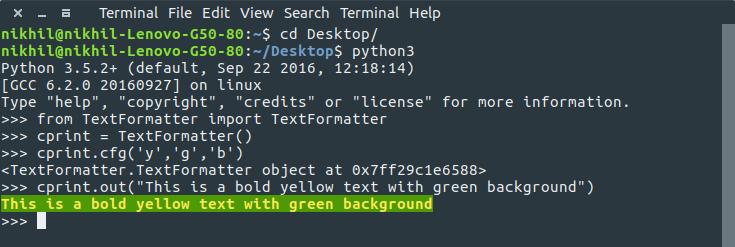GeeksforGeeks App
Open AppBrowser
Continue

# Formatted text in Linux Terminal using Python

This article demonstrates how to print formatted text in Linux terminal using Python programming language.

Formatted text(also called styled text or rich text) as opposed to plain text, has styling information like:

• color (text color, background color),
• style (bold or italic),
• and some other special features like Strike-through text, underlined text, hyperlinks, etc.

In a Linux Terminal, ANSI escape codes (or escape sequences) are used to control the formatting, color, and other output options.

To encode this formatting information, certain sequences of bytes are embedded into the text, which the terminal looks for and interprets as commands and executes them.

Let us go through a basic example of printing formatted text through a simple Python print statement!

 `print``(``'\x1b[3;31;43m'` `+` `'Hello world!'` `+` `'\x1b[0m'``)`

If you run this code on Linux Terminal, you will obtain an output like this:As you can see, the output has:

• text-color(foreground color): Red
• background color: Yellow
• text-style: Italic

Now, let us try to understand the meaning of the ANSI escape codes used in above print statement.

• First ANSI escape code used is:
``````\x1b[3;31;43m
``````

The general syntax of this code is:

``````\x1b[A;B;C
``````

Here,

• A: text formatting style. It can take any value from 1 to 9.
ValueStyle
1bold
2faint
3italic
4underline
7reverse
8hide
9strikethrough
• B: text color or foreground color. It can take any value form 30-37.
• C: background color. It can take any value form 40-47.
B(for text)C(for background)Color
3040black
3141red
3242green
3343yellow
3444blue
3545magenta
3646cyan
3747white
• At the end, we use this ANSI escape code:
``````\x1b[0m
``````

This is the code used to reset the color/style changes to default values.

Now, we create a class in Python to achieve required formatting systematically!

 `# A python class definition for printing formatted text on terminal.``# Initialize TextFormatter object like this:``# >>> cprint = TextFormatter()``#``# Configure formatting style using .cfg method:``# >>> cprint.cfg('r', 'y', 'i')``# Argument 1: foreground(text) color``# Argument 2: background color``# Argument 3: text style``#``# Print formatted text using .out method:``# >>> cprint.out("Hello, world!")``#``# Reset to default settings using .reset method:``# >>> cprint.reset()`` ` `class` `TextFormatter:`` ` `    ``COLORCODE ``=` `{``        ``'k'``: ``0``,  ``# black``        ``'r'``: ``1``,  ``# red``        ``'g'``: ``2``,  ``# green``        ``'y'``: ``3``,  ``# yellow``        ``'b'``: ``4``,  ``# blue``        ``'m'``: ``5``,  ``# magenta``        ``'c'``: ``6``,  ``# cyan``        ``'w'``: ``7`   `# white``    ``}`` ` `    ``FORMATCODE ``=` `{``        ``'b'``: ``1``,  ``# bold``        ``'f'``: ``2``,  ``# faint``        ``'i'``: ``3``,  ``# italic``        ``'u'``: ``4``,  ``# underline``        ``'x'``: ``5``,  ``# blinking``        ``'y'``: ``6``,  ``# fast blinking``        ``'r'``: ``7``,  ``# reverse``        ``'h'``: ``8``,  ``# hide``        ``'s'``: ``9``,  ``# strikethrough``    ``}`` ` `    ``# constructor``    ``def` `__init__(``self``):``        ``self``.reset()`` ` `    ``# function to reset properties``    ``def` `reset(``self``):``        ``# properties as dictionary``        ``self``.prop ``=` `{ ``'st'``: ``None``, ``'fg'``: ``None``, ``'bg'``: ``None` `}``        ``return` `self`` ` `    ``# function to configure properties``    ``def` `cfg(``self``, fg, bg``=``None``, st``=``None``):``        ``# reset and set all properties``        ``return` `self``.reset().st(st).fg(fg).bg(bg)`` ` `    ``# set text style``    ``def` `st(``self``, st):``        ``if` `st ``in` `self``.FORMATCODE.keys():``            ``self``.prop[``'st'``] ``=` `self``.FORMATCODE[st]``        ``return` `self`` ` `    ``# set foreground color``    ``def` `fg(``self``, fg):``        ``if` `fg ``in` `self``.COLORCODE.keys():``            ``self``.prop[``'fg'``] ``=` `30` `+` `self``.COLORCODE[fg]``        ``return` `self`` ` `    ``# set background color``    ``def` `bg(``self``,bg):``        ``if` `bg ``in` `self``.COLORCODE.keys():``            ``self``.prop[``'bg'``] ``=` `40` `+` `self``.COLORCODE[bg]``        ``return` `self`` ` `    ``# formatting function``    ``def` `format``(``self``, string):``        ``w ``=` `[``self``.prop[``'st'``],``self``.prop[``'fg'``], ``self``.prop[``'bg'``]]``        ``w ``=` `[ ``str``(x) ``for` `x ``in` `w ``if` `x ``is` `not` `None` `]``        ``# return formatted string``        ``return` `'\x1b[%sm%s\x1b[0m'` `%` `(``';'``.join(w), string) ``if` `w ``else` `string`` ` `    ``# output formatted string``    ``def` `out(``self``, string):``        ``print``(``self``.``format``(string))`

This is the class definition of our text formatter.

In order to use it, save above Python script as TextFormatter.py and open terminal in the same folder where this file is stored.

Then, run Python interpreter through the terminal and import TextFormatter.

Given below is a screen-shot of a sample run:Here,

• We import TextFormatter class from TextFormatter module using:
`from TextFormatter import TextFormatter`
• Then, create an object of TextFormatter class using:
`cprint = TextFormatter()`
• Configure formatting of text using cprint.cfg method. Here, argument 1 is text-color, argument 2 is background color and argument 3 is text style.
`cprint.cfg('y', 'g', 'b')`
• Now, simply print any statement using cprint.out method like this:
`cprint.out("Hello, world!")`

So, now, you can print styled text on terminal with ease!

This blog is contributed by Nikhil Kumar. If you like GeeksforGeeks and would like to contribute, you can also write an article using contribute.geeksforgeeks.org or mail your article to contribute@geeksforgeeks.org. See your article appearing on the GeeksforGeeks main page and help other Geeks.